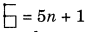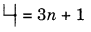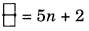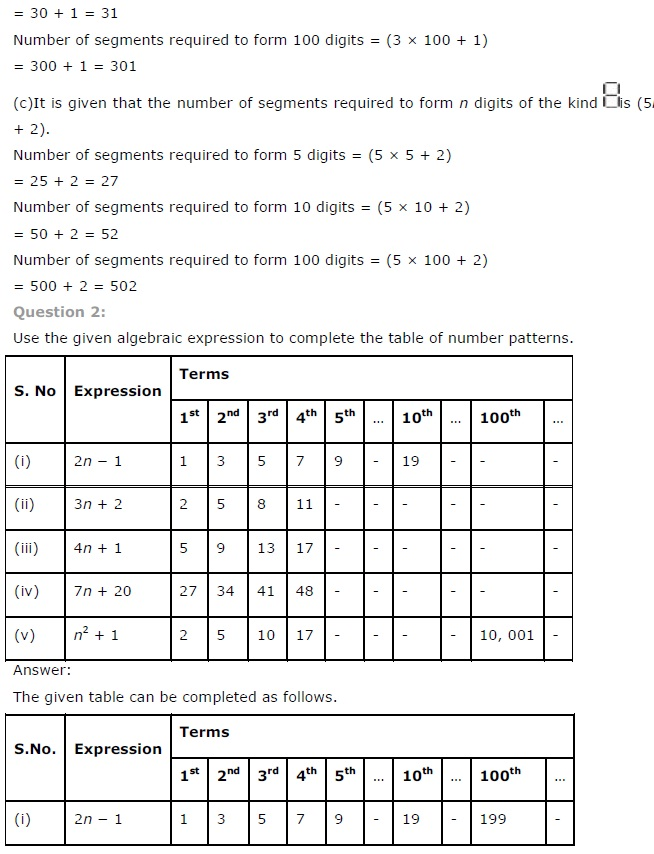# NCERT Solutions for Class 7 Maths Chapter 12 Algebraic Expressions Ex 12.4

NCERT Solutions for Class 7 Maths Chapter 12 Algebraic Expressions Exercise 12.4
Ex 12.4 Class 7 Maths Question 1.
Observe the patterns of digits made from line segments of equal length. You will find such segmented digits on the display of electronic watches or calculators.If the number of digits formed is taken to be n, the number of segments required to form n digits is given by the algebraic expression appearing on the right of each pattern. How many segments are required to form 5, 10, 100 digits of the kind 6, 4, 3.
Solution:
(i) The number of line segments required to form
n digits is given by the expressions.For 5 figures, the number of line segments = 5 × 5 + 1 = 25 + 1 = 26
For 10 figures, the number of line segments = 5 × 10 + 1
= 50 + 1 = 51
For 100 figures, the number of line segments = 5 × 100 + 1
= 500 + 1 = 501

(ii)For 5 figures, the number of line segments =3 ×5 + 1
= 15 + 1 = 16
For 10 figures, the number of line segments = 3 × 10 + 1
= 30 + 1 = 31
For 100 figures, the number of line segments = 3 × 100 + 1
= 300 + 1 = 301

(iii)For 5 figures, the number of line numbers = 5 × 5 + 2
= 25 + 2 = 27
For 10 figures, the number of line segments = 5 × 10 + 2
= 50 + 2 = 52
For 100 figures, the number of line segments = 5 × 100 + 2
= 500 + 2 = 502

Ex 12.4 Class 7 Maths Question 2.
Use the given algebraic expression to complete the table of number patterns:

 S.No. Expression Terms 1st 2nd 3rd 4th 5th … 10th … 100th … (i) 2n – 1 1 3 5 7 9 – 19 – – – (ii) 3n + 2 5 8 11 14 – – – – – – (iii) 4n + 1 5 9 13 17 – – – – – – (iv) 7n + 20 27 34 41 48 – – – – – – (v) n2 + 1 2 5 10 17 – – – – 10,001 –

Solution:
(i) Given expression is 2n – 1
For n = 100, 2 × 100 – 1
= 200 – 1 = 199

(ii) Given expression is 3n + 2
For n = 5, 3 × 5 + 2 = 15 + 2 = 17
For n = 10, 3 × 10 + 2 = 30 + 2 = 32
For n = 100, 3 × 100 + 2 = 300 + 2 = 302

(iii) Given expression is 4n + 1
For n = 5, 4 × 5 + 1 = 20 + 1 = 21
For n = 10, 4 × 10 + 1 = 40 + 1 = 41
For n = 100, 4 × 100 + 1 = 400 + 1 = 401

(iv) Given expression is 7n + 20
For n = 5, 7 × 5 + 20 = 35 + 20 = 55
For n = 10, 7 × 10 + 20 = 70 + 20 = 90
For n = 100, 7 × 100 + 20 = 700 + 20 = 720

(v) Given expression is n2 + 1
For n = 5, 52 + 1 = 25 + 1 = 26
For n = 10, 102 + 1 = 100 + 1 = 101+